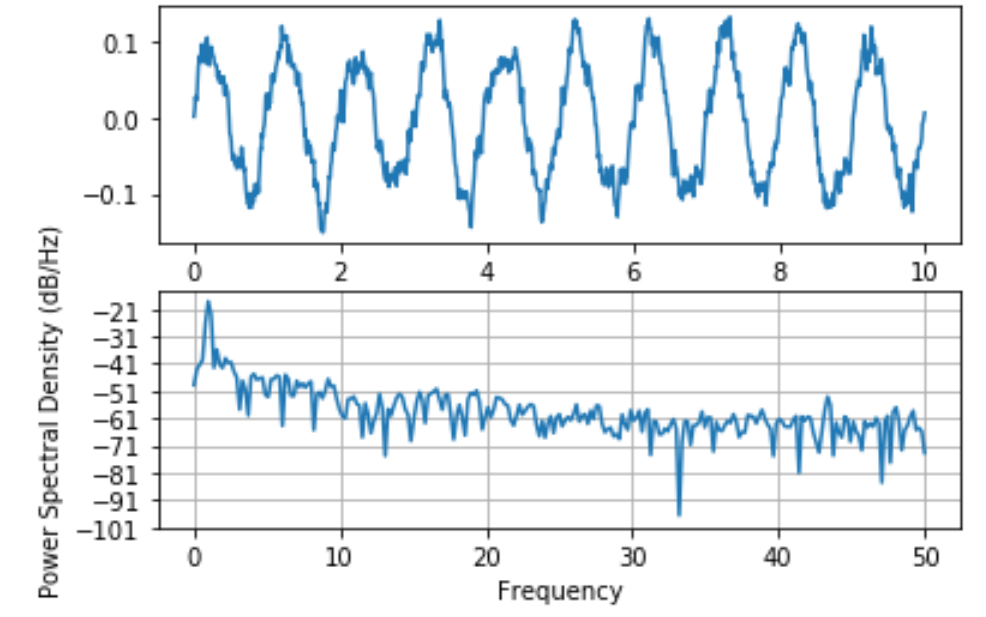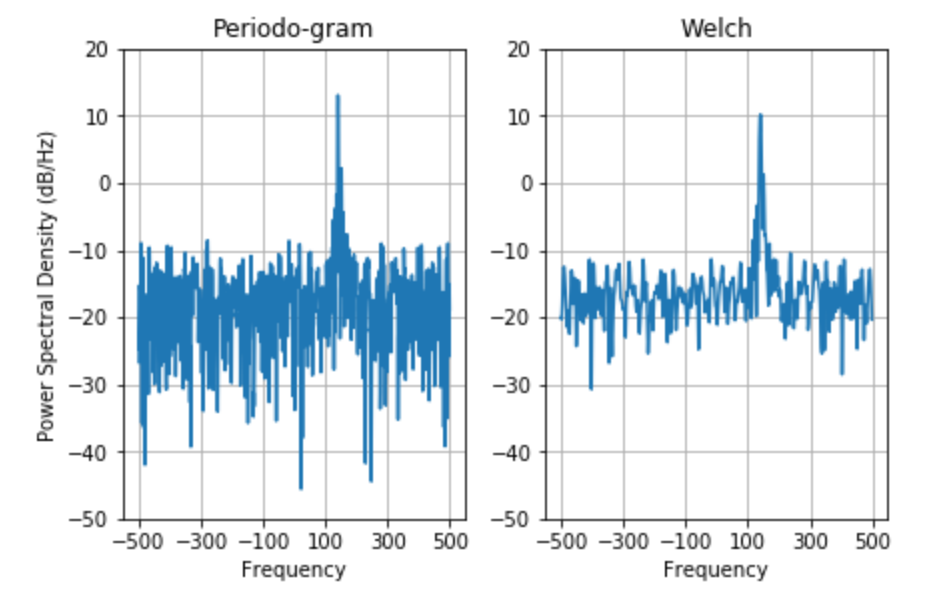# Plot the power spectral density using Matplotlib – Python

matplotlib.pyplot.psd() function is used to plot power spectral density. In the Welch’s average periodogram method for evaluating power spectral density (`say, Pxx`), the vector ‘x’ is divided equally into NFFT segments. Every segment is windowed by the function window and detrended by the function detrend. The overlap between segments is given by ‘noverlap’. The `|fft(i)|*2` of every segment ‘i’ are averaged together to compute P, with buffered scaling to correct for the power loss due to windowing.

Note: If len(x) < NFFT, it will be zero with NFFT padding.

Syntax: matplotlib.pyplot.psd(x, NFFT=None, Fs=None, Fc=None, detrend=None, window=None, noverlap=None, pad_to=None, sides=None, scale_by_freq=None, return_line=None, *, data=None, **kwargs)

Parameters:

1. x: It is a required parameter of 1D array or a sequence containing data
2. Fs: This is a required parameter that has a scalar value and has a default value of 2. Its value is the sampling frequency (samples per time unit). This value is used to calculate Fourier frequencies, freqs, in cycles per unit time.
3. window: This is a callable N-D array which is generally a function or a vector of NFFT length. Its default value is window_hanning. When a function is passed as a parameter/argument, it takes a data segment as an argument and returns the windowed version of the segment
4. sides: This parameter can have one of the three values namely ‘default’, ‘oneside’ or ‘twoside’. This is used for specifying which sides of the spectrum is to be returned. the ‘default’ gives its default behavior, which returns one-sided for real data and both for complex data. The ‘oneside’ value is used to force return a one-sided spectrum whereas the ‘twoside’ value is to return the two-sided spectrum.
5. >pad_to: This parameter holds an integer value tat represents the number of points on which the data segment is padded while performing the FFT. It is important to note that this is different from NFFT, which sets the number of data points used. This can give more points on the plot without increasing the actual resolution of the spectrum (the minimum distance between the resolvable peaks) allowing for more detail. This also corresponds to ‘n’ parameter in the call of fft(). Its default value is None that sets the pad_to equal to NFFT.
6. .NFFT: It holds an integer value representing the number of data points used in each block for FFT. The most efficient is the power of 2. Its default value is 256. Using this to get zero paddings must be avoided as it may result in incorrect scaling of the results, instead, pad_to is to be used for the same purpose.
7. detrend: It can accept three values namely ‘none’, ‘mean’, ‘linear’ or a callable and has default value as ‘none’.This function is designed to remove the mean or linear trend before fft-ing of each segment. The detrend in Matplotlib is a function unlike the detrend in MATLAB where it is a vector. The ‘detrend_none’, ‘detrend_mean’ and ‘detrend_linear’ are defined by the mlab module, but one can use custom function too. Strings can also choose a function. The ‘none’ calls ‘detrend_none’, the ‘linear’ calls ‘detrend_linear’ and the ‘mean’ calls the ‘detrend_mean’.
8. scale_by_freq: It is an optional argument that accepts a boolean value. It is used to specify whether the resulting density should be scaled by scaling the frequency. This would give the density in units of Hz^-1. This makes integration over the returned frequency values. The default for MATLAB compatibility is True.
9. noverlap: It is an integer value that represents the total number of points that overlap between segments. The default value for this is 0 suggesting no overlap.
10. Fc: It is an integer value representing the offsets of x extent of the plot to reflect the frequency range that is used when a signal is gained and then filtered after which it is downsampled to baseband. the ‘x’ represents the center frequency of x(default value of which is 0)
11. return_line: It is a boolean that decides if to include the line objected that is plotted in the return values. This value is False by default

Returns:

1. Pxx: It is a 1-D array which represents the the power spectrum P_{xx} before being scaled.
2. freqs: It is a !-D array represents the corresponding frequencies to the Pxx elements
3. line: It is a Line@D instance which is a line generated by the function. It only returns if return_line is set to True.

Other parameters:
**kwargs the keyword arguments are used to control the Line2D properties

Property Description
agg_filter a filter function that takes a (m, n, 3) float array and a dpi value that returns a (m, n, 3) array
alpha float
animated bool
antialiased or aa bool
clip_box Bbox
clip_on bool
clip_path [(Path, Transform)|Patch|None]
color or c color
contains callable
dash_capstyle {‘butt’, ’round’, ‘projecting’}
dash_joinstyle {‘miter’, ‘roung’, ‘bevel’}
dashes sequence of floats(on/off ink in points) or (None, None)
drawstyle or ds {‘default’, ‘steps’, ‘steps-pre’, ‘steps-mid’, ‘steps-post’}, default: ‘default’
figure figure
fillstyle {‘full’, ‘left’, ‘right’, ‘bottom’, ‘top’, ‘none’}
gid str
in_layout bool
label object
linestyle or lsl {‘-‘, ‘–‘, ‘-.’, ‘:’, ”, (offset, on-off-seq), …}
linewidth or lw float
marker marker style
markeredgecolor or mec color
Cmarkeredgewidth or mew float
markerfacecolor or mfc color
markerfacecoloralt or mfcalt color
markersize or ms float
markevery None or int or (int, int) or slice or List[int] or float or (float, float)
path_effects AbstractPathEffect
picker float or callable[[Artist, Event], Tuple[bool, dict]]
rasterized bool or None
sketch_params (scale: float, length: float, randomness: float)
snap bool or None
solid_capstyle {‘butt’, ’round’, ‘projecting’}
solid_joinstyle {‘miter’, ’round’, ‘bevel’}
transform matplotlib.transforms.Transform
url str
visible bool
xdata 1D array
ydata 1D array
zorder float

Example 1:

 `import` `matplotlib.pyplot as plt ` `import` `numpy as np ` `import` `matplotlib.mlab as mlab ` `import` `matplotlib.gridspec as gridspec ` ` `  `# set random state for reproducibility ` `np.random.seed(``19695601``) ` ` `  `diff ``=` `0.01` `ax ``=` `np.arange(``0``, ``10``, diff) ` `n ``=` `np.random.randn(``len``(ax)) ` `by ``=` `np.exp(``-``ax ``/` `0.05``) ` ` `  `cn ``=` `np.convolve(n, by) ``*` `diff ` `cn ``=` `cn[:``len``(ax)] ` `s ``=` `0.1` `*` `np.sin(``2` `*` `np.pi ``*` `ax) ``+` `cn ` ` `  `plt.subplot(``211``) ` `plt.plot(ax, s) ` `plt.subplot(``212``) ` `plt.psd(s, ``512``, ``1` `/` `diff) ` ` `  `plt.show() `

Output:Example 2:

 `import` `matplotlib.pyplot as plt ` `import` `numpy as np ` `import` `matplotlib.mlab as mlab ` `import` `matplotlib.gridspec as gridspec ` ` `  ` `  `# set random valueto ensure reproducibility ` `random_rep ``=` `np.random.RandomState(``19680801``)   ` ` `  `frame_per_second ``=` `1000` `a ``=` `np.linspace(``0``, ``0.3``, ``301``) ` `b ``=` `np.array([``2``, ``8``]).reshape(``-``1``, ``1``) ` `c ``=` `np.array([``150``, ``140``]).reshape(``-``1``, ``1``) ` `d ``=` `(b ``*` `np.exp(``2j` `*` `np.pi ``*` `c ``*` `a)).``sum``(axis ``=` `0``) ``+` `5` `*` `random_rep.randn(``*``a.shape) ` ` `  `figure, (a0, a1) ``=` `plt.subplots(ncols ``=` `2``, ` `                                ``constrained_layout ``=` `True``) ` ` `  `e ``=` `np.arange(``-``50``, ``30``, ``10``) ` `f ``=` `(e[``0``], e[``-``1``]) ` `g ``=` `np.arange(``-``500``, ``550``, ``200``) ` ` `  `a0.psd(d, NFFT ``=` `301``,  ` `       ``Fs ``=` `frame_per_second, ` `       ``window ``=` `mlab.window_none,  ` `       ``pad_to ``=` `1024``, ` `       ``scale_by_freq ``=` `True``) ` ` `  `a0.set_title(``'Periodo-gram'``) ` `a0.set_yticks(e) ` `a0.set_xticks(g) ` `a0.grid(``True``) ` `a0.set_ylim(f) ` ` `  `a1.psd(d, NFFT ``=` `150``, ` `       ``Fs ``=` `frame_per_second, ` `       ``window ``=` `mlab.window_none,  ` `       ``pad_to ``=` `512``,  ` `       ``noverlap ``=` `75``, ` `       ``scale_by_freq ``=` `True``) ` ` `  `a1.set_title(``'Welch'``) ` `a1.set_xticks(g) ` `a1.set_yticks(e) ` ` `  `# overwriting the y-label added by `psd` ` `a1.set_ylabel('') ` `a1.grid(``True``) ` `a1.set_ylim(f) ` ` `  `plt.show() `

Output:My Personal Notes arrow_drop_upCheck out this Author's contributed articles.

If you like GeeksforGeeks and would like to contribute, you can also write an article using contribute.geeksforgeeks.org or mail your article to contribute@geeksforgeeks.org. See your article appearing on the GeeksforGeeks main page and help other Geeks.

Please Improve this article if you find anything incorrect by clicking on the "Improve Article" button below.

Article Tags :

Be the First to upvote.

Please write to us at contribute@geeksforgeeks.org to report any issue with the above content.